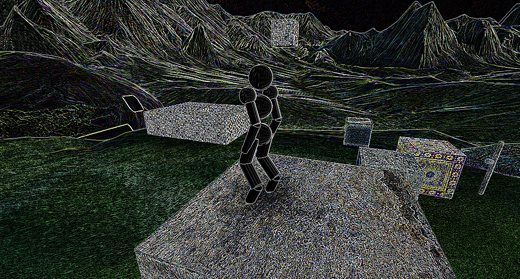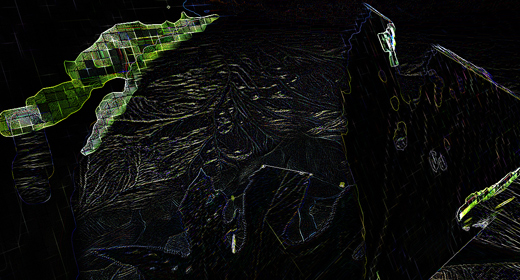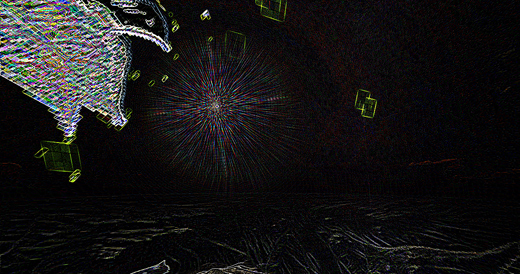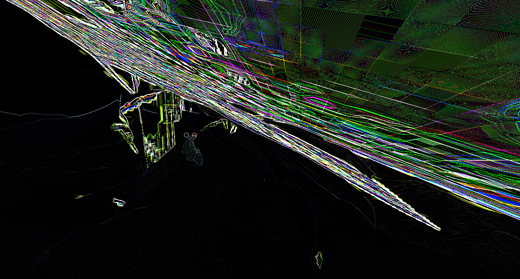# Post processing - part one

April 22nd, 2009

Inspired by recent blog comments about bloom, I added a post-processing step to the rendering pipeline in Overgrowth. Post processing effects are image filters that are applied as a final step before each rendered frame is drawn to the screen -- much like Photoshop filters. One of the more interesting effects I tested is edge detection: setting the value of each pixel to its original value minus the average of its neighbors. Here is the pseudocode for the GLSL shader:

```vec3 color = tex2D( tex, tex_coords.st ).rgb + tex2D( tex, tex_coords.st + vec2( 1, 0) ).rgb * -0.25 + tex2D( tex, tex_coords.st + vec2(-1, 0) ).rgb * -0.25 + tex2D( tex, tex_coords.st + vec2( 0, 1) ).rgb * -0.25 + tex2D( tex, tex_coords.st + vec2( 0,-1) ).rgb * -0.25;```

Normally, this looks kind of like this:However, in some circumstances it looks a lot more like some kind of modern art. We see a lot of strange things working on shaders, so I thought I would share these with you:Soon I'll do a video on some common 'next-gen' post-processing shaders like bloom and depth-of-field.